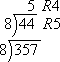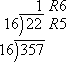UPSC  >  Introduction to Different Number Systems (Decimal, Binary, Octal, and Hexadecimal)

# Introduction to Different Number Systems (Decimal, Binary, Octal, and Hexadecimal) - UPSC Prelims Paper 2 CSAT - Quant, Verbal & Decision Making

Some important number systems are as follows.

• Decimal number system
• Binary number system
• Octal number system

Decimal number System

The Decimal Number System consists of ten digits from 0 to 9. These digits can be used to represent any numeric value. The base of decimal number system is 10. It is the most widely used number system. The value represented by individual digit depends on weight and position of the digit.

Let's take a closer look at something most of us take for granted.  Counting in decimal numbers.  For example, when you see the decimal number:

### 4275

it means that you sum the following to get a concept of the total quantity of things represented by that number:

 Thousands Hundreds Tens Ones 4 x 1000 plus    2 x 100 plus    7 x 10 plus    5 x 1

which is the same as:

 4 x 103 plus    2 x 102 plus    7 x 101 plus    5 x 100

(Remember that in mathematics, any number  raised to the zero power equals 1)

The number that is the on the far right is in the "ones" place holder (called the least significant digit).  To the left of that is the "tens" place holder.  To the left of that is the "hundreds" place holder, and so on.  They are all powers of 10.  Whatever digit is sitting in a particular place holder, you multiply that place holder amount ( power of 10) by the digit, and then add them all up.

 Thousands column (most significant digit) Hundreds column Tens column Ones column (least significant digit) 103 102 101 100 digit from 0-9 x 1000 digit from 0-9 x 100 digit from 0-9 x 10 digit from 0-9 x 1 4 2 7 5

= 4 thousand, 2 hundred, and seventy-five
= 4275

Binary Number System

Digital computer represents all kinds of data and information in the binary system. Binary Number System consists of two digits 0 and 1. Its base is 2. Each digit or bit in binary number system can be 0 or 1. A combination of binary numbers may be used to represent different quantities like 1001. The positional value of each digit in binary number is twice the place value or face value of the digit of its right side. The weight of each position is a power of 2.

In binary (Base-2), all the columns are powers of 2.  Think of the following table as the four least significant digit place holders for the binary number system just like the tables above using the decimal number system:

 Eights column (most significatnt digit) Fours column Twos column Ones column (least significant digit) 23 22 21 20

The far right colum is the "ones" place holder.  To the left of that is the "twos" place holder.  To the left of that is the "fours" place holder (22 = 4).  To the left of that is the "eights" place holder (23 = 8).  They are all powers of 2.  Whatever digit is sitting in a particular place holder, you multiply that place holder amount ( power of 2) by the digit, and then add them all up.  However, remember that a binary digit has only two options, 0 or 1, so it's very easy.

For example, let's look at the binary number:

### 1011

You would this  interpret in decimal as:

 Eights Fours Twos Ones 1 x 23 plus   0 x 22 plus   1 x 21 plus   1 x 20 which equals 1 x 8 plus   0 x 4 plus   1 x 2 plus   1 x 1 which equals 8 plus   0 plus   2 plus   1

The total is:  11 (in decimal)  which equals   8 + 0 + 2 + 1

In other words,

### 10112  =  1110

(Note: the subscripted number indicates the number base)

Octal Number System

Octal Number System consists of eight digits from 0 to 7. The base of octal system is 8. Each digit position in this system represents a power of 8. Any digit in this system is always less than 8. Octal number system is used as a shorthand representation of long binary numbers. The number 6418 is not valid in this number system as 8 is not a valid digit.

Let's dig right in:

• #### Convert 35710 to the corresponding base-eight number.

I will do the usual sequential division, this time dividing by 8 at each step:Once I got to the "5" on top, I had to stop, because 8 doesn't divide into 5.

Then the corresponding octal number is 5458.

• #### Convert 5458 to the corresponding decimal number.

I will follow the usual procedure, listing the digits in one row and then counting off the digits from the RIGHT in the next row, starting at zero:

 digits: 5 4 5 numbering: 2 1 0

Then I'll do the usual addition and multiplication:

5×82 + 4×81 + 5×80

= 5×64 + 4×8 + 5×1

= 320 + 32 + 5

= 357

Then the corresponding decimal number is 35710.

The Hexadecimal Number System consists of 16 digits from 0 to 9 and A to F. The alphabets A to F represent decimal numbers from 10 to 15. The base of this number system is 16. Each digit position in hexadecimal system represents a power of 16. The number 76416 is valid hexadecimal number. It is different from 76410 which is seven hundred and sixty four. This number system provides shortcut method to represent long binary numbers.

• #### Convert 35710 to the corresponding hexadecimal number.

Here, I will divide repeatedly by 16, keeping track of the remainders as I go. (You might want to use some scratch paper for this.)Reading off the digits, starting from the top and wrapping around the right-hand side, I see that:

35710 = 16516.

• #### Convert 16516 to the corresponding decimal number.

List the digits, and count them off from the RIGHT, starting with zero:

 digits: 1 6 5 numbering: 2 1 0

Remember that each digit in the hexadecimal number represents how many copies you need of that power of sixteen, and convert the number to decimal:

1×162 + 6×161 + 5×160

= 1×256 + 6×16 + 5×1

= 256 + 96 + 5

= 357

Then 16516 = 35710.

The document Introduction to Different Number Systems (Decimal, Binary, Octal, and Hexadecimal) | UPSC Prelims Paper 2 CSAT - Quant, Verbal & Decision Making is a part of the UPSC Course UPSC Prelims Paper 2 CSAT - Quant, Verbal & Decision Making.
All you need of UPSC at this link: UPSC

## FAQs on Introduction to Different Number Systems (Decimal, Binary, Octal, and Hexadecimal) - UPSC Prelims Paper 2 CSAT - Quant, Verbal & Decision Making

 1. What is the decimal number system?Ans. The decimal number system is a base-10 system that uses 10 digits (0-9) to represent numbers. It is the most commonly used number system in everyday life.
 2. What is the binary number system?Ans. The binary number system is a base-2 system that uses only two digits (0 and 1) to represent numbers. It is widely used in computer science and digital electronics.
 3. What is the octal number system?Ans. The octal number system is a base-8 system that uses 8 digits (0-7) to represent numbers. It is often used in computer programming, particularly when dealing with bitwise operations.
 4. What is the hexadecimal number system?Ans. The hexadecimal number system is a base-16 system that uses 16 digits (0-9 and A-F) to represent numbers. It is commonly used in computer science and programming, especially when dealing with memory addresses and binary data.
 5. How do you convert numbers between different number systems?Ans. To convert numbers between different number systems, you can use the following methods: - Decimal to binary: Divide the decimal number by 2 repeatedly and note the remainders. The binary representation is obtained by arranging the remainders in reverse order. - Binary to decimal: Multiply each binary digit by the corresponding power of 2 and sum the results. - Decimal to octal: Divide the decimal number by 8 repeatedly and note the remainders. The octal representation is obtained by arranging the remainders in reverse order. - Octal to decimal: Multiply each octal digit by the corresponding power of 8 and sum the results. - Decimal to hexadecimal: Divide the decimal number by 16 repeatedly and note the remainders. The hexadecimal representation is obtained by replacing each remainder greater than 9 with the corresponding letter (A-F) and arranging them in reverse order. - Hexadecimal to decimal: Multiply each hexadecimal digit by the corresponding power of 16 and sum the results.

## UPSC Prelims Paper 2 CSAT - Quant, Verbal & Decision Making

67 videos|50 docs|151 tests

## UPSC Prelims Paper 2 CSAT - Quant, Verbal & Decision Making

67 videos|50 docs|151 tests

### How to Prepare for UPSC

Read our guide to prepare for UPSC which is created by Toppers & the best Teachers
Signup to see your scores go up within 7 days! Learn & Practice with 1000+ FREE Notes, Videos & Tests.
10M+ students study on EduRev
Track your progress, build streaks, highlight & save important lessons and more!(Scan QR code)
Related Searches

,

,

,

,

,

,

,

,

,

,

,

,

,

,

,

,

,

,

,

,

,

,

,

,

,

,

,

,

,

,

,

,

,

;